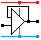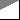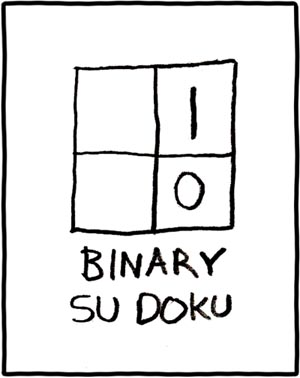Home

rOm
Quest
Glossary

Random
Page
Search
Site
Lush
Sim
Class
Subject
Images

Help
FAQ
Sign
Up
Log
In
 AS Level     >Basics Binary and Hex<

# Basics Binary and Hex

Site for Eduqas/WJEC - Go to the AQA site.

AS Level    Binary and HexadecimalQuestions 0 to 9   -->  View All

Binary is the base 2 number system counting 0, 1, 10, 11, 100, 101, 110, 111, 1000, etc.Thanks xkcd

## Decimal and Binary - Everyone

### Decimal Numbers

These are used in everyday life and are so familiar that many important features get forgotten.

• There are ten symbols. These are 0, 1, 2, 3, 4, 5, 6, 7, 8, 9.
• 9 + 1 = 10.
• 10 + 1 = 11.
• Counting from 0 to 9 is very familiar.
• For numbers greater than 9, a second column is needed.
• For numbers greater than 99, a third column is needed.
• The right hand column is the least significant. The numbers count in ones.
• The left hand column is the most significant. With three columns, the left hand column counts in hundreds.
• The Columns: 10000 - 1000 - 100 - 10 - 1 in decimal numbers.

### Binary Numbers

• There are two symbols. These are 0 and 1.
• 1 + 1 = 10.
• 10 + 1 = 11.
• There are 10 kinds of people. Those who understand binary and those who don't.
• Counting in binary: 0, 1, 10, 11, 100, 101, 110, 111, 1000 etc. Since there are only two symbols, you need to add a new column often.
• For numbers greater than 1, a second column is needed.
• For numbers greater than 11, a third column is needed.
• For numbers greater than 111, yet another column is needed.
• The right hand column is the least significant. The numbers count in ones.
• The left hand column is the most significant. With three columns, the left hand column counts in fours (in decimal numbers).
• The Columns: 128 - 64 - 32 - 16 - 8 - 4 - 2 - 1 in decimal numbers.

### Binary Bit

One binary digit. A zero or a one.

### Byte

A group of eight bits. Computer data is usually stored and processed in bytes or pairs of bytes or even groups of four or eight bytes.

### Nybble

This is half a byte or four bits. The word was originally a joke but the term proved useful.

In an eight bit byte such as 11100111, the HIGH nybble is 1110 and the LOW nybble is 0111.
They are called low and high because the low nybble is worth 0 to 15 in decimal numbers and the high nybble allows numbers up to 255.

Nybble is often spelled nibble. Internet flame wars rage over this!

### Word

Grouped bytes are called words. 64 bit computers common in 2015 work with 8 byte groups. 8 x 8 = 64 bits in the word.

### LSB and MSB

In this number: 11100111 the

• left most bit is worth 128 and is called the Most Significant Bit (MSB)
• right most bit is worth 1 and is called the Least Significant Bit (LSB)
• In a four bit number, the MSB is worth eight.

Getting bits back to front causes serious errors so it's important to pay attention to this.
This mistake crops up frequently when designing binary counters to reset on specific numbers like ten.

### Decimal Binary Conversion

• The columns from left to right are worth 8, 4, 2 and 1.
• Column 8 is the MSB or most significant bit. Column 1 if the LSB or least significant bit.
• The binary counts from 0 to 15 and the zeros and ones indicate the presence or absence of the 8, 4, 2 or 1 depending on which column they are in.
• For example 0101 means No 8, yes 4, no 2 and yes 1.
• Add them up and you get 4 + 1 = five.
 Decimal Binary 8 4 2 1 0 0 0 0 0 1 0 0 0 1 2 0 0 1 0 3 0 0 1 1 4 0 1 0 0 5 0 1 0 1 6 0 1 1 0 7 0 1 1 1 8 1 0 0 0 9 1 0 0 1 10 1 0 1 0 11 1 0 1 1 12 1 1 0 0 13 1 1 0 1 14 1 1 1 0 15 1 1 1 1

### Converting Larger Numbers

• Jot down a table like this with the column values. Write the binary number below the column headings.
• Add up the numbers wherever there is a binary one to get the total.
• To do a decimal to binary conversion, work from left to right and ask if you need each number. If you do, write a one. If not, write a zero.
 Decimal 128 64 32 16 8 4 2 1 Binary 0 0 1 1 0 0 0 1 Yes/No 0 0 32 16 0 0 0 1 Total = 49

### Little and Big Endian

A 64 bit computer could put the most significant byte at the left or right end of the group of eight bytes. Different computer manufacturers use both schemes. This makes it more difficult to store and transfer data between incompatible systems. Data munging is needed to reverse the byte order. Internet flame wars rage over this topic.

• There are sixteen symbols. These are 0, 1, 2, 3, 4, 5, 6, 7, 8, 9, A, B, C, D, E, F.
• 9 + 1 = A.
• F + 1 = 10.
• 10 + 1 = 11.
• Counting in hexadecimal: 0, 1 ... 8, 9, A, B ... E, F, 10, 11 ... 18, 19, 1A, 1B ... 1E, 1F, 20, 21, 22 ... 99, 9A, 9B ...FE, FF, 100
• For numbers greater than F, a second column is needed.
• For numbers greater than FF, a third column is needed.
• The right hand column is the least significant. The numbers count in ones.
• The left hand column is the most significant. With three columns, the left hand one counts in 256s (in decimal numbers).
• The Columns: 4096 - 256 - 16 - 1 in decimal numbers.

### Uses of Each System

• Humans use Decimal for normal everyday counting.
• Computers and digital systems use Binary.
• Computer programmers and electronic engineers use hexadecimal as a convenient shorthand for binary.
• Binary Coded Decimal is a convenient way for computers to represent/store digits that are going to be displayed.

It does not take long to jot down this table. Then you can just look up the binary/hexadecimal conversions

 Decimal Binary 8 4 2 1 0 0 0 0 0 1 0 0 0 1 2 0 0 1 0 3 0 0 1 1 4 0 1 0 0 5 0 1 0 1 6 0 1 1 0 7 0 1 1 1 8 1 0 0 0 9 1 0 0 1 A 1 0 1 0 B 1 0 1 1 C 1 1 0 0 D 1 1 0 1 E 1 1 1 0 F 1 1 1 1

### Converting Bigger Numbers

• To convert 11001010011100, first rewrite it in nybbles. A nybble is half a byte.
• You may need to add a few zeros to the left end of the number to get whole nybbles.
• You get 0011 - 0010 - 1001 - 1100.
• Now look up the table above and read off the hexadecimal digits.
 0011 0010 1001 1100 3 2 9 C

Here are some examples of hexadecimal numbers ...

 0x3F9C This notation is used by AQA, Microsoft Windows, C, C++, C# and Java programmers. &H3F9C This notation is used by BASIC programmers \$3F9C This notation is used by Pascal programmers 3F9C16 This is how hexadecimal numbers are written in printed text.

### Problems Solved Using 2N or 2N - 1

 What is the biggest number that can be stored in a six bit memory location? 26 - 1 = 63 If each pixel uses 24 bits to store the colour, how many colours are there? 224 = 16.7 Million A computer has a 20 bit address bus. How many addresses can it access? 220 = 1 Million A digital to analogue converter uses 8 bits. How many levels are available for the digitised signal? 28 = 256 A microcontroller has a 14 bit word. 8 bits are used for data and six for instruction op-codes. How many op-codes could be available? 26 = 64 How many colours are available if four but colour is being used? 24 = 16 What is the biggest number that can be stored in a four bit latch? 24 - 1 = 15 Monochrome displays use black and white. How many bits are needed to store each pixel? 21 = 2 so only one bit is needed.

Subject Name     Level     Topic Name     Question Heading     First Name Last Name Class ID     User ID

 CloseEscapeX Q: qNum of last_q     Q ID: Question ID         Score: num correct/num attempts         Date Done

Question Text

image url

Help Text
Debug

• You can attempt a question as many times as you like.
• If you are logged in, your first attempt, each day, is logged.
• To improve your scores, come back on future days, log in and re-do the questions that caused you problems.
• In the grade book, you can delete your answers for a topic before re-doing the questions. Avoid deleting unless you intend re-doing the questions very soon.